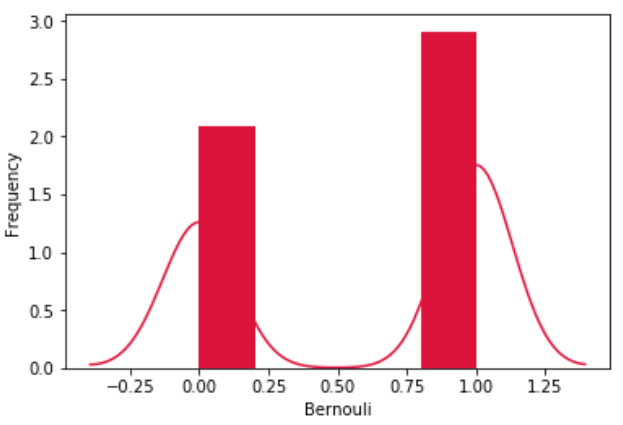# (40)Python伯努利分布

``````from scipy.stats import bernoulli
import seaborn as sb

data_bern = bernoulli.rvs(size=1000,p=0.6)
ax = sb.distplot(data_bern,
kde=True,
color='crimson',
hist_kws={"linewidth": 25,'alpha':1})
ax.set(xlabel='Bernouli', ylabel='Frequency')
```Python```关注右侧公众号，随时随地查看教程
Python数据分析教程目录